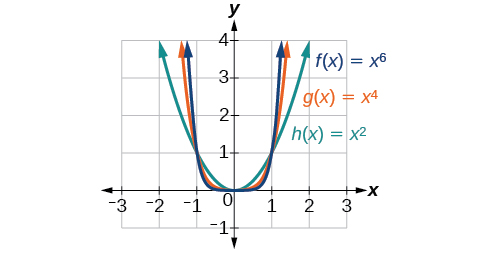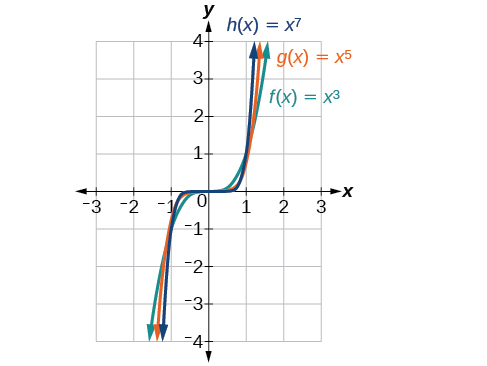# 5.2 Power functions and polynomial functions  (Page 2/19)

 Page 2 / 19Even-power functions

To describe the behavior as numbers become larger and larger, we use the idea of infinity. We use the symbol $\text{\hspace{0.17em}}\infty \text{\hspace{0.17em}}$ for positive infinity and $\text{\hspace{0.17em}}\mathrm{-\infty }\text{\hspace{0.17em}}$ for negative infinity. When we say that “ $x\text{\hspace{0.17em}}$ approaches infinity,” which can be symbolically written as $\text{\hspace{0.17em}}x\to \infty ,\text{\hspace{0.17em}}$ we are describing a behavior; we are saying that $\text{\hspace{0.17em}}x\text{\hspace{0.17em}}$ is increasing without bound.

With the positive even-power function, as the input increases or decreases without bound, the output values become very large, positive numbers. Equivalently, we could describe this behavior by saying that as $\text{\hspace{0.17em}}x\text{\hspace{0.17em}}$ approaches positive or negative infinity, the $\text{\hspace{0.17em}}f\left(x\right)\text{\hspace{0.17em}}$ values increase without bound. In symbolic form, we could write

[link] shows the graphs of $\text{\hspace{0.17em}}f\left(x\right)={x}^{3},\text{\hspace{0.17em}}g\left(x\right)={x}^{5},$ and $\text{\hspace{0.17em}}h\left(x\right)={x}^{7},$ which are all power functions with odd, whole-number powers. Notice that these graphs look similar to the cubic function in the toolkit. Again, as the power increases, the graphs flatten near the origin and become steeper away from the origin.Odd-power functions

These examples illustrate that functions of the form $\text{\hspace{0.17em}}f\left(x\right)={x}^{n}\text{\hspace{0.17em}}$ reveal symmetry of one kind or another. First, in [link] we see that even functions of the form even, are symmetric about the $\text{\hspace{0.17em}}y\text{-}$ axis. In [link] we see that odd functions of the form  odd, are symmetric about the origin.

For these odd power functions, as $\text{\hspace{0.17em}}x\text{\hspace{0.17em}}$ approaches negative infinity, $\text{\hspace{0.17em}}f\left(x\right)\text{\hspace{0.17em}}$ decreases without bound. As $\text{\hspace{0.17em}}x\text{\hspace{0.17em}}$ approaches positive infinity, $\text{\hspace{0.17em}}f\left(x\right)\text{\hspace{0.17em}}$ increases without bound. In symbolic form we write

The behavior of the graph of a function as the input values get very small ( $\text{\hspace{0.17em}}x\to -\infty \text{\hspace{0.17em}}$ ) and get very large ( $\text{\hspace{0.17em}}x\to \infty \text{\hspace{0.17em}}$ ) is referred to as the end behavior    of the function. We can use words or symbols to describe end behavior.

[link] shows the end behavior of power functions in the form $\text{\hspace{0.17em}}f\left(x\right)=k{x}^{n}\text{\hspace{0.17em}}$ where $\text{\hspace{0.17em}}n\text{\hspace{0.17em}}$ is a non-negative integer depending on the power and the constant.

Given a power function $\text{\hspace{0.17em}}f\left(x\right)=k{x}^{n}\text{\hspace{0.17em}}$ where $\text{\hspace{0.17em}}n\text{\hspace{0.17em}}$ is a non-negative integer, identify the end behavior.

1. Determine whether the power is even or odd.
2. Determine whether the constant is positive or negative.
3. Use [link] to identify the end behavior.

## Identifying the end behavior of a power function

Describe the end behavior of the graph of $\text{\hspace{0.17em}}f\left(x\right)={x}^{8}.$

The coefficient is 1 (positive) and the exponent of the power function is 8 (an even number). As $\text{\hspace{0.17em}}x\text{\hspace{0.17em}}$ approaches infinity, the output (value of $\text{\hspace{0.17em}}f\left(x\right)\text{\hspace{0.17em}}$ ) increases without bound. We write as $\text{\hspace{0.17em}}x\to \infty ,f\left(x\right)\to \infty .\text{\hspace{0.17em}}$ As $\text{\hspace{0.17em}}x\text{\hspace{0.17em}}$ approaches negative infinity, the output increases without bound. In symbolic form, as We can graphically represent the function as shown in [link] .

## Identifying the end behavior of a power function.

Describe the end behavior of the graph of $\text{\hspace{0.17em}}f\left(x\right)=-{x}^{9}.$

The exponent of the power function is 9 (an odd number). Because the coefficient is $\text{\hspace{0.17em}}–1\text{\hspace{0.17em}}$ (negative), the graph is the reflection about the $\text{\hspace{0.17em}}x\text{-}$ axis of the graph of $\text{\hspace{0.17em}}f\left(x\right)={x}^{9}.\text{\hspace{0.17em}}$ [link] shows that as $\text{\hspace{0.17em}}x\text{\hspace{0.17em}}$ approaches infinity, the output decreases without bound. As $\text{\hspace{0.17em}}x\text{\hspace{0.17em}}$ approaches negative infinity, the output increases without bound. In symbolic form, we would write

#### Questions & Answers

A laser rangefinder is locked on a comet approaching Earth. The distance g(x), in kilometers, of the comet after x days, for x in the interval 0 to 30 days, is given by g(x)=250,000csc(π30x). Graph g(x) on the interval [0, 35]. Evaluate g(5)  and interpret the information. What is the minimum distance between the comet and Earth? When does this occur? To which constant in the equation does this correspond? Find and discuss the meaning of any vertical asymptotes.
Kaitlyn Reply
The sequence is {1,-1,1-1.....} has
amit Reply
circular region of radious
Kainat Reply
how can we solve this problem
Joel Reply
Sin(A+B) = sinBcosA+cosBsinA
Eseka Reply
Prove it
Eseka
Please prove it
Eseka
hi
Joel
June needs 45 gallons of punch. 2 different coolers. Bigger cooler is 5 times as large as smaller cooler. How many gallons in each cooler?
Arleathia Reply
7.5 and 37.5
Nando
find the sum of 28th term of the AP 3+10+17+---------
Prince Reply
I think you should say "28 terms" instead of "28th term"
Vedant
the 28th term is 175
Nando
192
Kenneth
if sequence sn is a such that sn>0 for all n and lim sn=0than prove that lim (s1 s2............ sn) ke hole power n =n
SANDESH Reply
write down the polynomial function with root 1/3,2,-3 with solution
Gift Reply
if A and B are subspaces of V prove that (A+B)/B=A/(A-B)
Pream Reply
write down the value of each of the following in surd form a)cos(-65°) b)sin(-180°)c)tan(225°)d)tan(135°)
Oroke Reply
Prove that (sinA/1-cosA - 1-cosA/sinA) (cosA/1-sinA - 1-sinA/cosA) = 4
kiruba Reply
what is the answer to dividing negative index
Morosi Reply
In a triangle ABC prove that. (b+c)cosA+(c+a)cosB+(a+b)cisC=a+b+c.
Shivam Reply
give me the waec 2019 questions
Aaron Reply

### Read also:

#### Get the best Algebra and trigonometry course in your pocket!

Source:  OpenStax, Algebra and trigonometry. OpenStax CNX. Nov 14, 2016 Download for free at https://legacy.cnx.org/content/col11758/1.6
Google Play and the Google Play logo are trademarks of Google Inc.

Notification Switch

Would you like to follow the 'Algebra and trigonometry' conversation and receive update notifications?ByByByByBy Mariah HauptmanByBy